## Example Questions

### Example Question #41 : Integers

Add: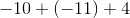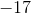Explanation:

Simply the signs before solving. A positive sign multiplied with a negative sign will convert the sign to a negative, and a negative multiplied with a negative will convert the sign to a positive.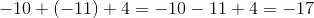### Example Question #42 : Integers

Solve: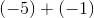Explanation:

Starting atand addingis the same as subtracting.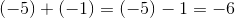.

### Example Question #43 : Integers

Solve: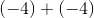Explanation:

Adding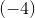tois the same as subtractingfrom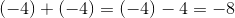.

### Example Question #44 : Integers

Add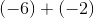Starting atand addingis the same as subtracting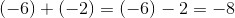.